# Excel Data Analysis Function That You Can Try

• Last Updated : 07 Oct, 2021

Have you ever analyze any data? What does it mean? Well, analyzing any kind of data means interpreting, collecting, transforming, cleaning, and visualizing data to discover valuable insights that drive smarter and more effective decisions related to business or anywhere you need it. So, Excel solves a big problem as you can use functions to analyze your data.

In Excel, there are around 475 functions for analyzing a data set. It becomes so hard to remember each and every function in Excel. Sometimes people tried to used calculation manually rather than calculating on Excel as using many functions becomes complicated to them. Thus, there are some important Excel Data Analysis Functions that you can try, and that will help you a lot.

Attention reader! Don’t stop learning now. If you are an Excel beginner (or an intermediate) and want to learn Excel, Geeksforgeeks brings the perfect course for you to start, Diving Into Excel

Let’s start learning the Function!

## Excel Data Analysis Function That You Can Try

Whether you are a Data analyst or someone who uses Excel for calculation or for small business, These functions for everyone. These functions will ease your data analyst task:

### CONCATENATE Function

This is the easiest function to understand but the most useful one. It will combine text, numbers, dates from multiples cells into one. This will helpful for analyzing data also helps at the time of creating JAVA queries.

Formula for using CONCATENATE function:

` =CONCATENATE(text1,[text2], [text3]...)`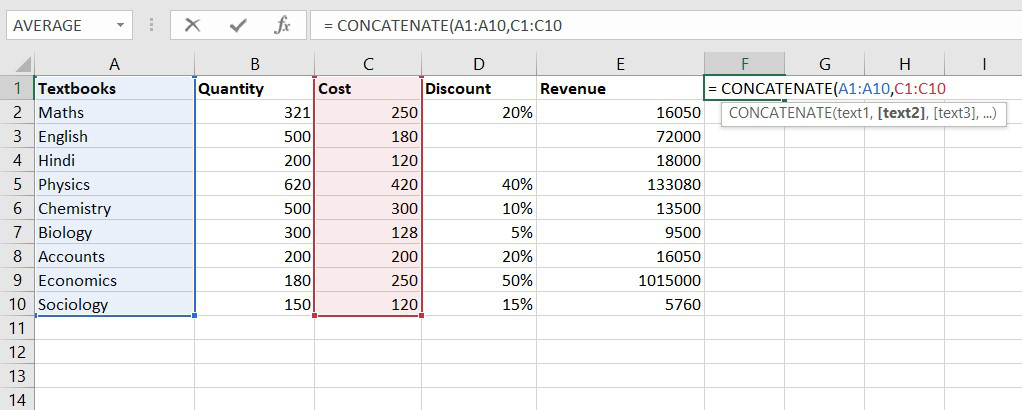Example of  Data Analysis Function

### COUNTA Function

The COUNTA function counts the number of cells that are not blank, it counts a number of cells whether it contains a text, number or an error message. But, keep in mind COUNTA function does not count empty cell.

Formula for COUNTA function :

`= COUNT(value1, [value2], …)`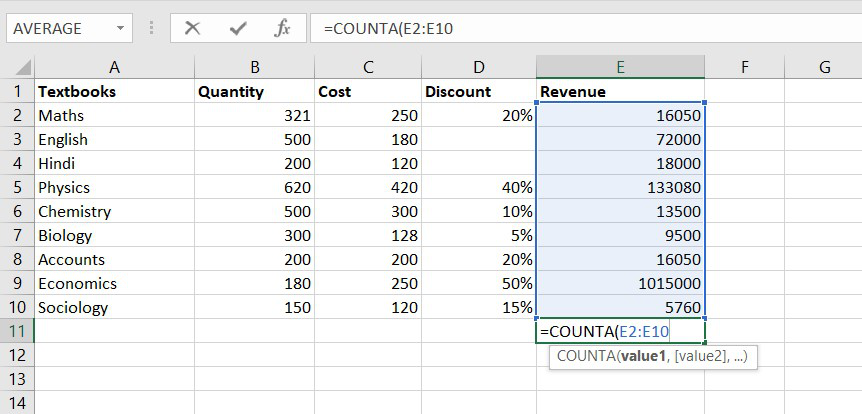Example of  Data Analysis Function

### DAYS Function

DAYS function used to find the number of days between two calendar dates. This is a very helpful analyzing function. Let’s look at the formula.

Formula for DAYS function:

`= DAYS(end_date, start_date)`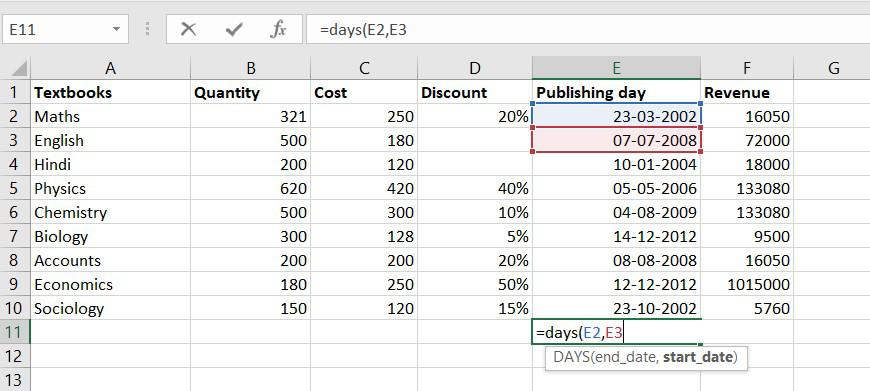Example of  Data Analysis Function

### NETWORKDAYS Function

The NETWORKDAYS function is a date-time function used to calculate the number of working days between two calendar dates. It will exclude weekends and holidays.

Formula for DAYS function:

`= DAYS(end_date, start_date)`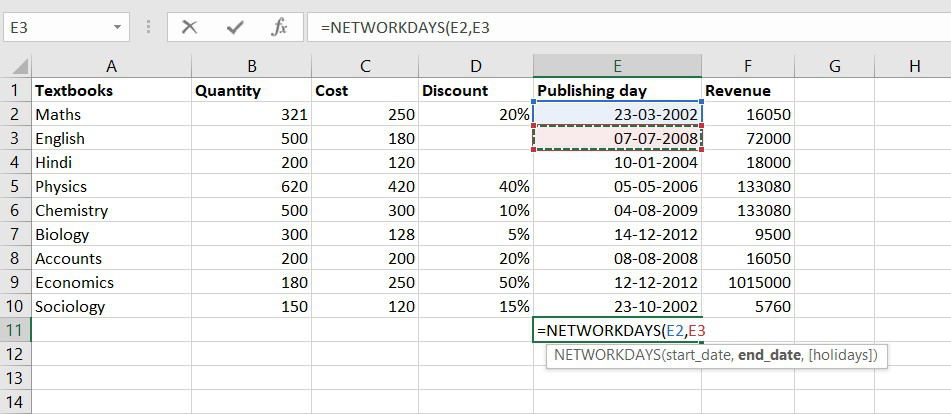Example of  Data Analysis Function

### SUMIFS Function

The function will work on one or more than one condition in a given range and adds all the cell arguments that will meet the conditions.

Formula for SUMIFS function:

`= SUMIFS(sum_range, criteria_range1, criteria1, [criteria_range2, criteria2], ...)`

### AVERAGEIFS Function

The function will calculate the average of all the cells that will meet multiple conditions.

Formula for SUMIFS function:

`=AVERAGEIF (range, criteria, [average_range])`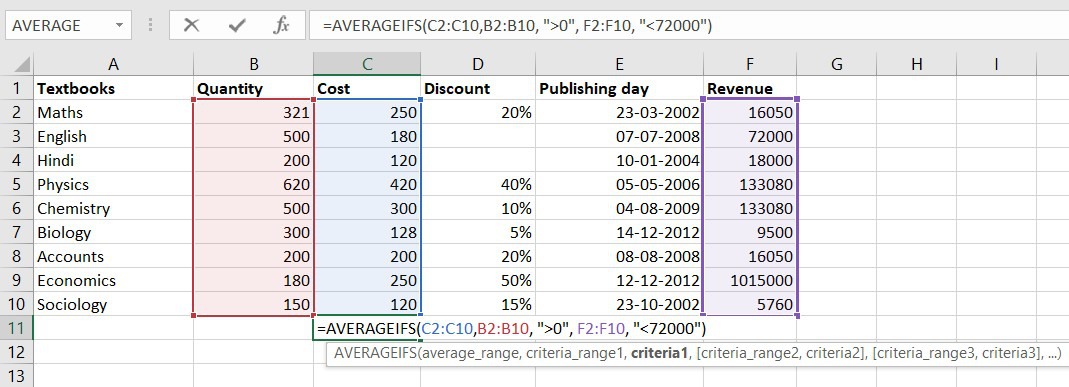Example of  Data Analysis Function

### SEARCH Function

This function is again a very powerful tool when you deal with big data. You can find any data entry through this function. One important thing to note there is a difference between the FIND and SEARCH function, as FIND is used for case-sensitive matches only but SEARCH will find both types of words (capitals and small).

Formula for SEARCH function:

`=SEARCH(find_text, within_text, [start_num])`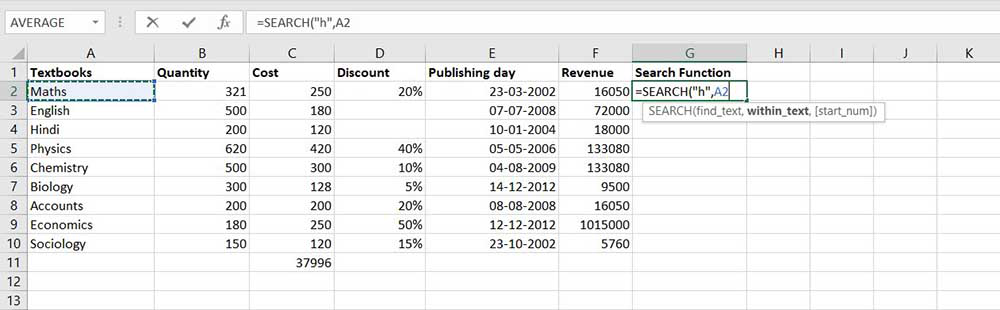Example of  Data Analysis Function

### VLOOKUP Function

Basically, VLOOKUP function stands for Vertical Lookup, so the function will find the specific value in the column and in order to return a value from a different column in the same row.

Formula for VLOOKUP function:

`= (lookup_value, table_array, col_index_num, [range_lookup])`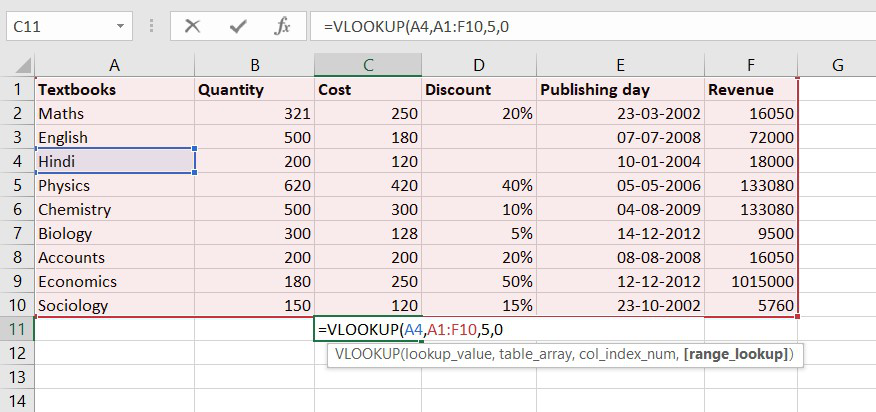Example of  Data Analysis Function

### COUNTIFS Function

The COUNTIFS function is the most used function in Excel. The function will work on one or more than one condition in a given range and counts the cell that meets the condition.

Formula for COUNTIFS function:

`= COUNTIFS (range1, criteria1, [range2], [criteria2], ...)`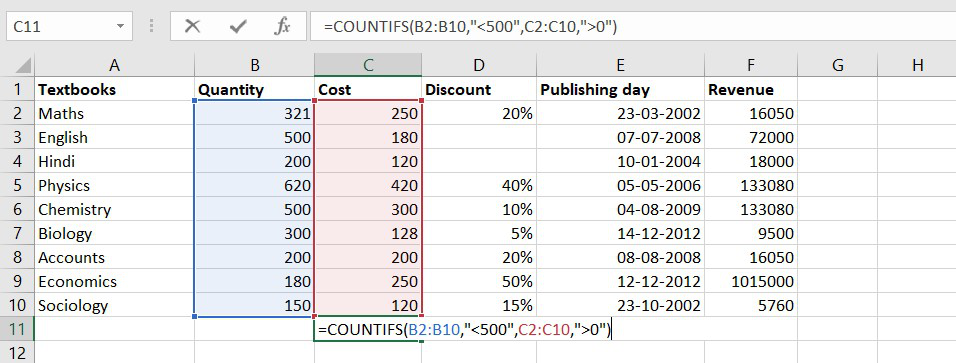Example of  Data Analysis Function

### SUMPRODUCT Function

It is an inbuilt function in Excel, it will return the sum of products of the value given in the argument or an array. This function usually uses when you need to multiply many cells together.

Formula for SUMPRODUCT function:

`= SUMPRODUCT ( array1, [array2],[array3],...)`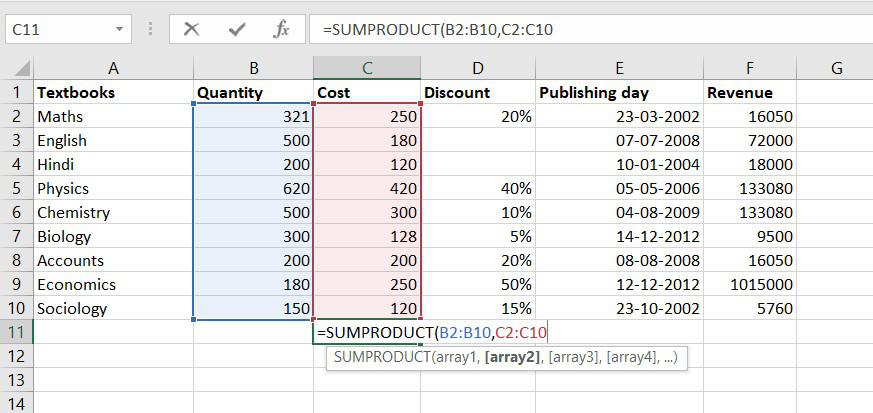Example of  Data Analysis Function

My Personal Notes arrow_drop_up